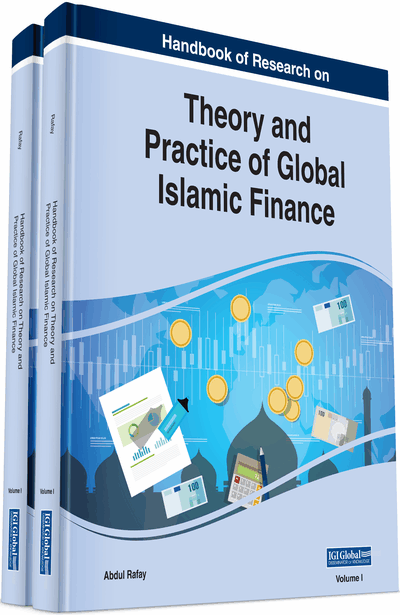Positive Interest Rate, Stationary Economy, and Inefficiency

Amir Kia (Utah valley University, USA)
DOI: 10.4018/978-1-7998-0218-1.ch001
Available
\$37.50
No Current Special Offers

Abstract

This chapter analyses the direct impact of a positive rate of interest (usury) on the production possibility curve. Usury under a stationary state creates inefficiency in the sense that the marginal rate of transformation is not equal to the price ratio. Over the short run Pareto efficiency appears when a transition period is considered and the rate of return moving from one state to another is endogenous and equals the rate of investment. In a non-stationary economy, when a positive rate of return (interest) is equal to the growth rate of the economy, there will be a Pareto-efficient equilibrium. But if the interest rate is exogenous to the system, usury exists, and then Pareto efficiency cannot be achieved under any state, either stationary or non-stationary.
Chapter Preview
Top

Background

Numerous studies show that the existence of a predetermined positive rate of interest will lead to Pareto inefficiency in a stationary economy. However, in a timeless production system, a non-zero predetermined rate of interest cannot create inefficiency in a Pareto sense, that is, the cost minimization process under a competitive system leads to the equality of marginal rate of transformation and the price ratio. On the other hand, a time-phased system, both Ricardian and non-Ricardian, can be proved to be Pareto-efficient when the predetermined rate of interest is zero under perfect competition. M-S in their time-phased neo-Ricardian system proved that the existence of a predetermined positive rate of interest in a stationary state creates inefficiency in an economic system under perfect competition. The M-S proposition was studied by Samuelson (1975) who admitted that the existence of a predetermined positive rate of interest, under a stationary state, pushes the production possibility curve of the world inward. He also claimed that a positive rate of interest acts as distorting taxes. He contended that the intertemporal efficiency standpoint in a closed economy does not create a Pareto-efficient situation. Ethier (1979) also refers to the M-S model and questions how the rate of interest is exogenous when capital goods are supplied endogenously. For Dixit (1981), the stationary assumption is only a special instance of general equilibrium over time, and very far from reality. Pareto inefficiency was not followed in the existing literature. This chapter fills the gap.

Complete Chapter List

Search this Book:
Reset